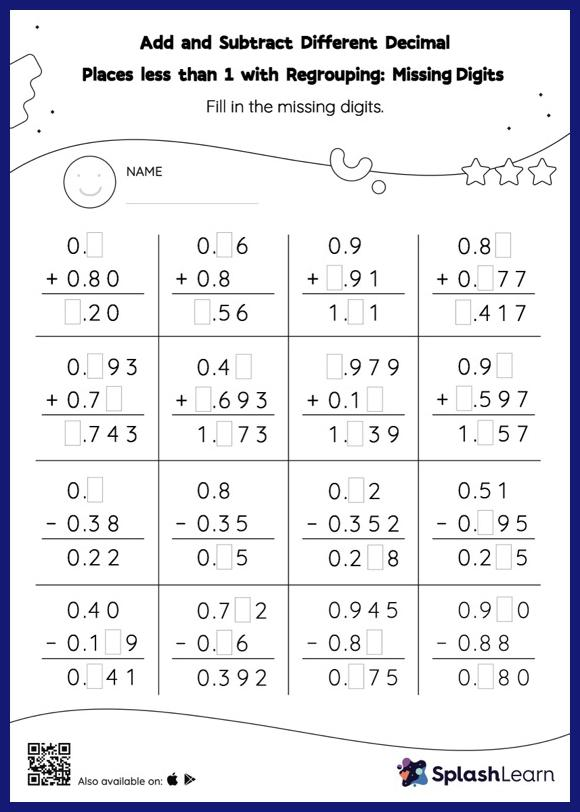# Add and Subtract Different Decimal Places less than 1 with Regrouping: Missing Digits Worksheet

Home > Add and Subtract Different Decimal Places less than 1 with Regrouping: Missing DigitsWhile adding and subtracting decimals, students use the relationship between addition and subtraction to find the missing number. They also need to regroup numbers in add and subtract different decimal places less than 1 with regrouping worksheet utilizing the relationship between ones, tenth, hundredth, thousandth, etc., To get to the result. As the worksheet uses the column method, it is helpful in getting students toward higher accuracy, especially with bigger numbers and in scenarios where regrouping is required.